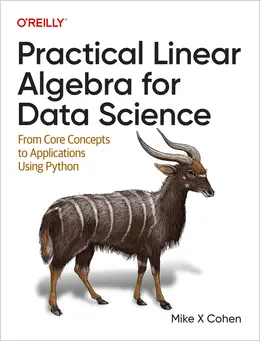# Practical Linear Algebra for Data Science### eBook Details:

• Paperback: 328 pages
• Publisher: WOW! eBook (October 18, 2022)
• Language: English
• ISBN-10: 1098120612
• ISBN-13: 978-1098120610

### eBook Description:

Practical Linear Algebra for Data Science: From Core Concepts to Applications Using Python

If you want to work in any computational or technical field, you need to understand linear algebra. As the study of matrices and operations acting upon them, linear algebra is the mathematical basis of nearly all algorithms and analyses implemented in computers. But the way it’s presented in decades-old textbooks is much different from how professionals use linear algebra today to solve real-world modern applications.

Ideal for practitioners and students using computer technology and algorithms, this Practical Linear Algebra for Data Science book introduces you to:

• The interpretations and applications of vectors and matrices
• Matrix arithmetic (various multiplications and transformations)
• Independence, rank, and inverses
• Important decompositions used in applied linear algebra (including LU and QR)
• Eigendecomposition and singular value decomposition
• Applications including least-squares model fitting and principal components analysis

This practical guide from Mike X Cohen teaches the core concepts of linear algebra as implemented in Python, including how they’re used in data science, machine learning, deep learning, computational simulations, and biomedical data processing applications. Armed with knowledge from this book, you’ll be able to understand, implement, and adapt myriad modern analysis methods and algorithms.